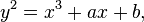# {{theTime}}

## Total Pageviews

### What is Elliptic curve cryptography

Elliptic curve cryptography (ECC) is an approach to public-key cryptography based on the algebraic structure of elliptic curves over finite fields. The use of elliptic curves in cryptography was suggested independently by Neal Koblitz and Victor S. Miller in 1985.
Elliptic curves are also used in several integer factorization algorithms that have applications in cryptography, such as Lenstra elliptic curve factorization.

Public-key cryptography is based on the intractability of certain mathematical problems. Early public-key systems are secure assuming that it is difficult to factor a large integer composed of two or more large prime factors. For elliptic-curve-based protocols, it is assumed that finding the discrete logarithm of a random elliptic curve element with respect to a publicly known base point is infeasible. The size of the elliptic curve determines the difficulty of the problem. The primary benefit promised by ECC is a smaller key size, reducing storage and transmission requirements—i.e., that an elliptic curve group could provide the same level of security afforded by an RSA-based system with a large modulus and correspondingly larger key—e.g., a 256bit ECC public key should provide comparable security to a 3072bit RSA public key (see #Key sizes).
For current cryptographic purposes, an elliptic curve is a plane curve which consists of the points satisfying the equation$y^2 = x^3 + ax + b, \,$
along with a distinguished point at infinity, denoted ∞. (The coordinates here are to be chosen from a fixed finite field of characteristic not equal to 2 or 3, or the curve equation will be somewhat more complicated.)
This set together with the group operation of the elliptic group theory form an Abelian group, with the point at infinity as identity element. The structure of the group is inherited from the divisor group of the underlying algebraic variety.
As for other popular public key cryptosystems, no mathematical proof of security has been published for ECC as of 2009. However, the U.S. National Security Agency has endorsed ECC by including schemes based on it in its Suite B set of recommended algorithms and allows their use for protecting information classified up to top secret with 384-bit keys. While the RSA patent expired in 2000, there are patents in force covering certain aspects of ECC technology, though some[who?] argue that the Federal elliptic curve digital signature standard (ECDSA; NIST FIPS 186-3) and certain practical ECC-based key exchange schemes (including ECDH) can be implemented without infringing them.